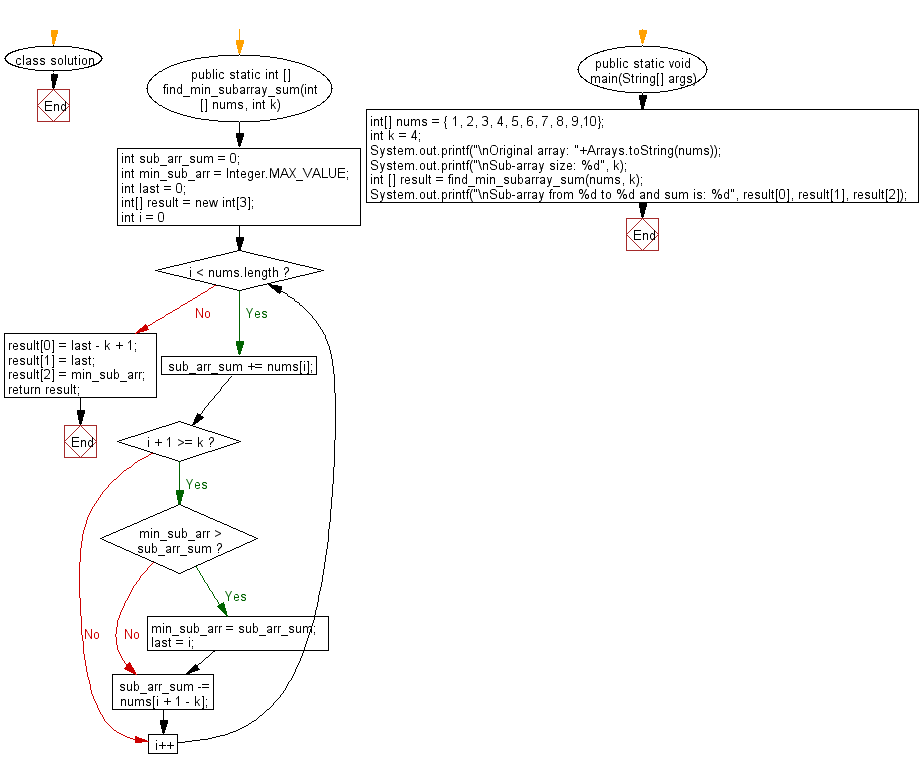﻿ Java: Minimum subarray sum of specified size in an array# Java Array Exercises: Find minimum subarray sum of specified size in a given array of integers

## Java Array: Exercise-69 with Solution

Write a Java program to find minimum subarray sum of specified size in a given array of integers.

Example:
Input :
nums = { 1, 2, 3, 4, 5, 6, 7, 8, 9,10}
Output:
Sub-array size: 4
Sub-array from 0 to 3 and sum is: 10

Sample Solution:

Java Code:

``````import java.util.*;
class solution {

public static int [] find_min_subarray_sum(int[] nums, int k)
{
int sub_arr_sum = 0;
int min_sub_arr = Integer.MAX_VALUE;
int last = 0;
int[] result = new int;

for (int i = 0; i < nums.length; i++)
{
sub_arr_sum += nums[i];

if (i + 1 >= k)
{
if (min_sub_arr > sub_arr_sum)
{
min_sub_arr = sub_arr_sum;
last = i;
}

sub_arr_sum -= nums[i + 1 - k];
}
}
result = last - k + 1;
result = last;
result = min_sub_arr;
return result;
}

public static void main(String[] args)
{
int[] nums = { 1, 2, 3, 4, 5, 6, 7, 8, 9,10};
int k = 4;
System.out.printf("\nOriginal array: "+Arrays.toString(nums));
System.out.printf("\nSub-array size: %d", k);
int [] result = find_min_subarray_sum(nums, k);
System.out.printf("\nSub-array from %d to %d and sum is: %d", result, result, result);
}
}
```
```

Sample Output:

```Original array: [1, 2, 3, 4, 5, 6, 7, 8, 9, 10]
Sub-array size: 4
Sub-array from 0 to 3 and sum is: 10
```

Flowchart:Java Code Editor:

Improve this sample solution and post your code through Disqus

What is the difficulty level of this exercise?

Test your Programming skills with w3resource's quiz.

﻿

## Java: Tips of the Day

countOccurrences

Counts the occurrences of a value in an array.

Use Arrays.stream().filter().count() to count total number of values that equals the specified value.

```public static long countOccurrences(int[] numbers, int value) {
return Arrays.stream(numbers)
.filter(number -> number == value)
.count();
}
```

Ref: https://bit.ly/3kCAgLb